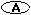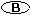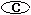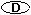Forces and Motion Theme Unit edHelper.com subscribers: Click Here to Build a New Printable Version of this Pre-Made Worksheet with Answers! Not a subscriber?  Sign up now for the subscriber materials! Return to Forces and Motion Theme Unit

 Name _____________________________Date ___________________
Select the definition that most nearly defines the given word.
Sample
This is only a sample pre-made worksheet. Sign up now!

1.   motionThe natural force produced by a magnetic field.A measure of the force of gravity on an object.A device used for measuring weight.A change in the position or place of something over time in comparison to a reference point.
2.   frictionA unit that is a measure of force.The unit of work in the metric system.Energy in the form of a push or a pull.A force that opposes motion between two surfaces that are touching.
3.   workA measure of how fast something moves over a distance.A measure of the amount of force needed to move an object a certain distance.The rate at which velocity changes.A measurement of the motion of something. This is equal to the product of the moving object's mass times its velocity.
4.   inertiaA device used for measuring mass.A force that pulls objects towards each other.A measure of both the speed and direction of a moving object.The tendency of an object to resist a change in motion.
5.   weightThe amount of matter that an object has.A measure of the force of gravity on an object.A force that opposes motion between two surfaces that are touching.A measure of the amount of force needed to move an object a certain distance.
6.   jouleA measure of how fast something moves over a distance.The natural force produced by a magnetic field.A device used for measuring weight.The unit of work in the metric system.
7.   massA measure of the force of gravity on an object.The rate at which velocity changes.The amount of matter that an object has.A force that pulls objects towards each other.
8.   balanceA measure of both the speed and direction of a moving object.A measure of the amount of force needed to move an object a certain distance.A device used for measuring mass.A change in the position or place of something over time in comparison to a reference point.
9.   magnetismThe natural force produced by a magnetic field.A force that opposes motion between two surfaces that are touching.A device used for measuring mass.A unit that is a measure of force.
10.   spring scaleEnergy in the form of a push or a pull.A device used for measuring weight.The unit of work in the metric system.The tendency of an object to resist a change in motion.
11.   accelerationEnergy in the form of a push or a pull.A measurement of the motion of something. This is equal to the product of the moving object's mass times its velocity.The unit of work in the metric system.The rate at which velocity changes.
12.   velocityThe rate at which velocity changes.A measure of both the speed and direction of a moving object.A measure of how fast something moves over a distance.A force that opposes motion between two surfaces that are touching.